# Implicit differentiation

## Summary and examples

Often a function is given in explicit form, that is to say in the form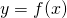. When we want to differentiate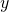towe use the table of standard functions or the product, quotient or chain rule, or a combination of these methods.

It also occurs that a function is not in explicit form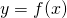but in implicit form, that is to say in the form. In this case we might want to define a derivative of, but we do not know immediately how to do this. We discuss this topic here.

In this context, it is useful to realize that we would have to actually write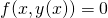. After all,is a function of. But mostly (mathematicians are often lazy) we writeinstead or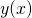which is a shorthand notation.
To show how to differentiate an implicit function, we look at some examples.

##### Example 1

Determine the derivative ofwhen this is given in explicit form: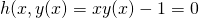We do not have the functionavailable in explicit form (which can be found quite easily in this case, see later)), we need to apply implicit differentiation.

We notice that the formula which has to be differentiated is a product of two functions of. We call one function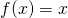and the other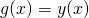. Now we apply the product rule: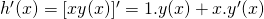So we can write: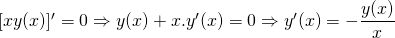Unfortunately, the derivative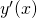is available as a function ofwhich we do not know.

As mentioned earlier, the implicit expression: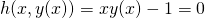can also be written explicitly: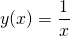When we substitutein the derivative above, we get: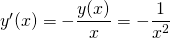This a result which we would have expected.

##### Example 2

The following example (as more examples) is taken from the book Sydsaeter and Hammond: Essential Mathematics for Economic Analysis (4th ed).

Determine the derivative in the point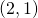of the graph of the implicitly given function of: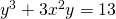We notice that it is not easy (and maybe impossible) to deduce an explicit functionfrom this implicit function.

Yet we want to find the derivative in the given point, and thus we have to use implicit differentiation.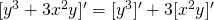The second term is not difficult to differentiate. The first term requires some explanation. We do not have to differentiateitself – this would yield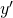- but a function ofnamely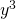. In such cases we have to apply the chain rule: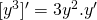Just to understand this, look at a similar example. We know that the derivative of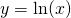equals, and thus, when we want to differentiate the derivative of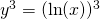than applying the chain rule yields: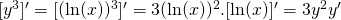.

So we get: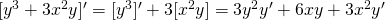and have to solvefrom the equation: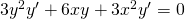which results in the derivative: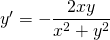Thus, in the pointwe find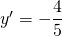##### Example 3

The following formula describes the graph of a circle with center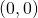and radius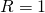: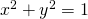In which points of the circle does the tangent line have a slope equal to(meaning that the angle between tangent line and-axis is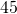degrees)?

In order to find the slope we have to differentiate the implicit function: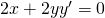orA slope equal tomeans that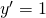or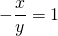or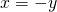.
If we combine this with the equationwe find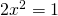, so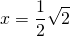or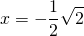.
The points we have been looking for are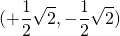), or.
Just check this in the figure of the circle.

0